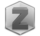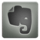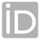You are in:Home/Publications/Performance Evaluation of Applying Fuzzy Multiple Regression Model to TLS in the Geodetic Coordinate Transformation

### Prof. Amr Hanafi Ahmed Ali :: Publications:

 Title: Performance Evaluation of Applying Fuzzy Multiple Regression Model to TLS in the Geodetic Coordinate Transformation Authors: Amr H. Ali; Mona S. ElSayed Year: 2016 Keywords: Transformation; weighted least squares; weighted total least squares; singular value decomposition; Linear Regression; Fuzzy Multiple Regression Model. Journal: American Scientific Research Journal for Engineering, Technology, and Sciences Volume: 25 Issue: 1 Pages: 36 - 50 Publisher: Not Available Local/International: International Paper Link: Not Available Full paper Amr Hanafi Ahmed Ali_2074-5988-1-PB(1).pdf Supplementary materials Not Available
 Abstract: It is known that the classical technique for solving the linear regression problem of the geodetic transformation process is using least squares approach (LS). On the contrary, this research explores the application of total least squares (TLS) approach to solve linear regression with and without fuzzy multiple regression model in Bursa-Wolf similarity transformation process. In this research two groups of data sets are used; the first group is the solution points which are used to compute the values of the transformation parameters. The second is the check points that were used to assess the accuracy of the applied methods (in terms of mean and Root Mean Squares Errors RMSE). The applied four solutions show how the accuracy of TLS is relatively better than LS. The weight has a better effect on improving the accuracy of both cases, LS and TLS; however, its effects are greater on TLS. By using the fuzzy multiple regression models, the results improved further and the need for accurate weights/confidence is eliminated.